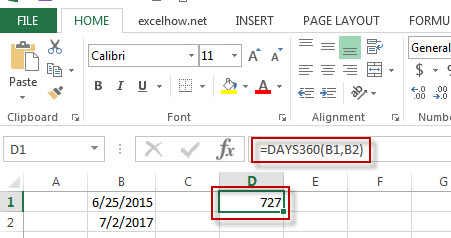# Excel Days360 Function

This post will guide you how to use Excel DAYS360 function with syntax and examples in Microsoft excel.

### Description

The Excel DAYS360 function will calculate the number of days between two dates based on a 360-day year.

The DAYS360 function is a build-in function in Microsoft Excel and it is categorized as a  DATE and TIME Function.

The DAYS360 function is available in Excel 2016, Excel 2013, Excel 2010, Excel 2007, Excel 2003, Excel XP, Excel 2000, Excel 2011 for Mac.

### Syntax

The syntax of the DAYS360 function is as below:

=DAYS360 (start_date, end_date,[method])

Where the DAYS360 function arguments are:
Start_date and end_date -This is a required argument. The two dates to calculate the number of days.
Method – This is an optional argument.  It’s a Boolean value. If TRUE is set, the DAYS360 function will use the European method. Or it will use US method.

### Example

The below examples will show you how to use Excel DAYS360 Function to return the number of days between two dates based on a 360-day year.

#1 =DAYS360(B1,B2)Note: the above excel formula returns the number of days between 6/25/2015 and 7/2/2017, based on a 360-day year.